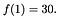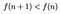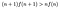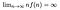# My first bonding curve

The text below used to live as a pdf on my little academic website a while ago. In 2014, I was tutoring a few students and I started wondering about how to scale my services. I wanted to know if you could manipulate prices (in a fair way) to incentivize students to spread the word of my services. Could I come up with a pricing mechanism that would benefit my tutees and also work in my favour?

I re-read this a few months back and showed it to a friend. He said that this was essentially a bonding curve. I hadn’t thought about that — I never actually drew the curve. So, I copy-and-paste the original text below. I think it’s a cute little read and could also potentially show what a bonding curve is (or at least what it attempts to achieve) in a first principled manner.From the wikipedia article on the Harmonic series

# Introduction

I currently tutor a couple of students, two of which are taking the same course. I likely won’t have the time to tutor any more students individually. So I am considering offering group tutoring sessions. Since I don’t advertise myself and rely solely on word-of-mouth, I need something to incentivize my current tutees to continue letting other students know of my tutoring services (since they likely prefer individual/small group tutoring sessions). This is simple: offer each student a lower price; however, since I want to increase my profits (of course), there is only so much I can lower the price per student

# The Problem

How much do I charge each student? More formally: let n be the number of students I tutor per session. Let f be the amount I charge each student per hour. Then, nf(n) is the amount I make per hour. Now, the question becomes: what should f be?

# The Minimum Requirements

The function f must satisfy the following set of properties:(1). Since \$30/hour is how much I currently charge per student.(2). Each student should be charged less as the number of students increases.(3). The amount I make per hour should increase as the number of students increases.(4). Obviously — I’d like to theoretically make infinite profits from my tutoring services.

# The solution

My intuition led me immediately to the harmonic sequence:

Letting f(n) = 1/n would satisfy property (2), and “almost” (3). Some experimentation uncovered:

This satisfies all properties. Since the limit of f(n) as n goes to is B, we can consider B to be some sort of “base price”. I settled on B =15 … for now. And so I have:

Under this function, tutoring one, two, three, four students at a time will have me profit \$30, \$45, \$60, \$75 an hour, respectively (where the students will be charged \$30, \$22.5, \$20, \$18.75, respectively). Win-win I’d say!

The above started off as a practical question but it ended up as a theoretical exercise instead. That is, I never did end up implementing this pricing scheme; I just had a fun time thinking about the problem. I also remember thinking the formula was such an obvious thing and that I must have come across something that already exists somewhere in economics literature. I guess not. But the concept is now very well-entrenched in the cryptosphere as something called a bonding curve.

Software Engineer, Data Scientist, Co-founder @ API3